# Modules of methods of calculation¶

## Tight-Binding method¶

Semiempirical Tight Binding method was realized.

class kvazar.core.tb.TB(struct, parm)[source]

Class that realizes Tight-Binding method.

Constructor of class TB.

Args:

struct (Structure): nanostructure.

lib (module): dynamic library, it realize filling Hamiltonian matrix, calculating repulsive energie, forces acting on atoms.

parms (dict): parameters of method.

Kwargs:

functions (list): list of functions that will be loaded from lib library.
compute()[source]

Calculating full energy of nanostructure.

Returns:

energy_total(float): full energy of nanostructure
compute_ebond()[source]

Calculating bond energy using formula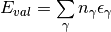where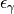is a value on diagonal of diagonalised hamiltonian

Returns:

energy_bond(float): bond energy of atoms
compute_erep()[source]

Repulsive energy between two atoms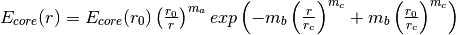Total repulsive energy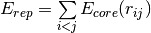Returns:
energy_rep(float): repulsive energy
ensure_forces()[source]

Function changes method for calculating forces, acting on atoms of structure, to ensure, that hamiltonian matrix is not zero before calculation start and eigenvectors can be calculated.

Loading of function that calculate forces and creating wrap-methods from dynamic library.

Args:

functions (list): list of functions, that will be loaded from lib

lib (module): dynamic library

Loading of functions from dynamic library and creating wrap-methods.

Args:

functions (list): list of functions, that will be loaded from lib

lib (module): dynamic library

preprocessing(struct)[source]

Preprocessing for TB method, that:

1. Checks existing of parameters for different atom types, involved in structure.
2. Creates Hamiltonian matrix.
3. Calculates number of orbitals of each type and shift indices for orbitals in hamiltonian.
4. Calculates number of occupied energy levels
Args:
struct (Structure): nanostructure
1. Updates data of tight bonds in structure
2. Zeros hamiltonian matrix
Args:

tb_links(numpy.array): 2D arrays of bonds.

num_tb_links(numpy.array): 1D array, it contains numbers of bonds for each atom

## Molecular mechanics¶

Molecular mechanics method was realized.

class kvazar.core.mm.MM(struct, lib, ff_functions, ff_params)[source]

Wrap class for computational modules of molecular mechanical method.

Constructor of class:

Args:

struct(structure): nanostructure

lib(module): dynamic library

ff_functions(list): list of names of force field functions

ff_params(list): list of names of parameters of force field functions

compute()[source]

Returns energy of structure

compute_i(i)[source]

Returns summ of energies, calculated by functions from list ‘functions_i’

compute_ij(i, j)[source]

Returns summ of energies, calculated by functions from list ‘functions_ij’

Adds function ‘forces’ to module

Args:

lib(module): dynamic library

Args:

ff_functions(list): list of names of atom types

lib(module): dynamic library for molecular mechanics computation

preprocessing(struct)[source]

It checks matching of atom types and atom names of structure with the same parameters of force field. If there are no atom types in structure function rebuilds it from atom names. If there are no atom names, it prints, that it’s not possible to check out correctness of atom types.

## Reactive empirical bond order potential¶

Method REBO was realised according to article: Donald W. Brenner, Olga A. Shenderova, Judith A. Harrison, Steven J. Stuart, Boris Ni, and Susan B. Sinnott, “A second-generation reactive empirical bond order (REBO) potential energy expression for hydrocarbons” // J. Phys.: Condens. Matter 14(2002), P. 783–802. Description for method was taken from this article.

Chemical binding energy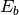can be written as a sum over nearest neighbours in the form:

(1)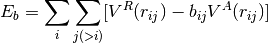The equation (1) is used for the total potential energy. Following the earlier hydrocarbon bonding expression, the empirical bond order function used here is written as a sum of terms:

(2)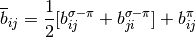Values for the function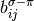and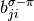depend on the local coordination and bond angles for atomsand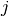respectively. The function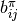is further written as a sum of two terms:

(3)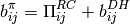The first generation hydrocarbon expression used Morse-type terms for the pair interactions in equation (1). It was determined that this form is too restrictive to simultaneously fit equilibrium distances, energies, and force constants for carbon-carbon bonds. This form has the further disadvantage that both terms go to finite values as the distance between atoms decreases, limiting the possibility of modeling process involving energetic atomic collisions. In the second-generation potential next forms are used for attractive ad repulsive potentials:

(4)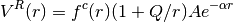and

(5)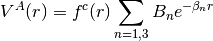Analytic forms for each term in equation (2) are fitted to both the discrete values of the bond orders above, and to other properties of solid-state and molecular carbon as described below. The first term in equation (2):

(6)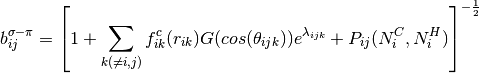where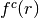is a function to ensure that interations include only nearest neighbours. P represents a bicubic spline and quantities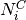and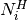represent the number of carbon and hydrogen atoms, respectively, that are neighbours of atom. It’s defined by next equations:

(7)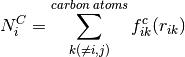and

(8)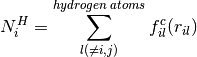The term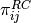is taken as tricubic spline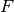:

(9)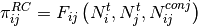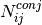is given by next expression:

(10)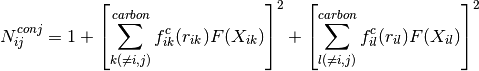where

(11)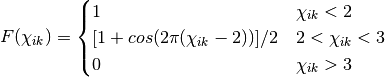and

(12)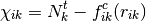The term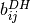in equation (3) :

(13)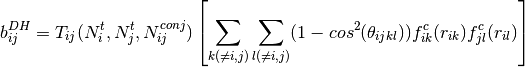where

(14)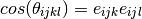The function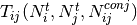is a tricubic spline,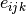and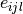are unit vectors in the direction of the cross products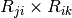and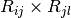, respecrively, where R are vectors connecting subscripted atoms.

The value ofis defined by a switching function of the form:

(15)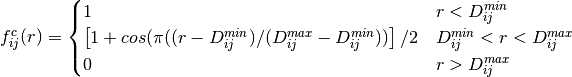## Adaptive intermolecular reactive empirical bond order potential¶

AIREBO method was realized according to article: Steven J. Stuart, Alan B. Tutein and Judith A. Harrison. “A reactive potential for hydrocarbons with intermolecular interactions” // Journ. of Chem. Phys., V 112,14 (2000), p. 6472-6486.

AIREBO potential can be represented by a sum over pairwise interactions, including covalent bonding REBO interactions, LJ terms, and torsion interactions:

(16)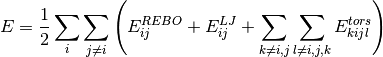Van-der-Vaals interaction is described through the using of the Lennard-Jones potential:

(17)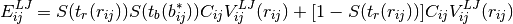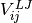is the traditional LJ term:

(18)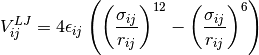It was modified by several sets of switching functions. S(t) can be represented in the next form:

(19)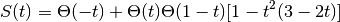Magnitude of LJ interactions depends on bonding environment. Gradual exclusion of Lennard-Jones interactions with changings of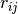is controlled by scalling function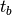:

(20)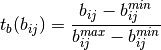If atomsandare not connected by two or fewer intermediate atoms, LJ interactions between them are controlled by next switching function:

(21)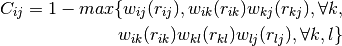where: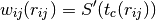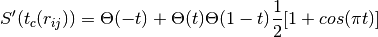The torsional part of equation (16) for the dihedral angle determined by atoms i, j, k, l has the next form:

(22)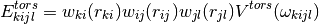where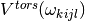is the torsional potential:

(23)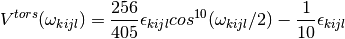class kvazar.core.airebo.AIREBO(struct, ff_params, comm=None)[source]

This class is the successor of class MM

Constructor of class AIREBO:

Args:

struct(structure): nanostructure

ff_params(list): list of names of potentials functions

init_cutoff(r_in, r_out, cutoff_neighbours)[source]

Args:

r_in(float): internal cutoff radius

r_out(float): external cutoff radius

cutoff_neighbours(list): list of neighbours get into the field with r_out radius

preprocessing(struct)[source]

It corrects atom type and atom boxnames, group them and find out. Masses are taken from parameters.

update_cutoff(cutoff_neighbours)[source]

Updates list of neighbours in cutoff area

Args:

cutoff_neighbours(list): list of neighbours

## Coarse-grained modeling¶

MARTINI force field for coarse-grained modeling was realized.

class kvazar.core.martini.MARTINI(struct, ff_params)[source]

This class is the successor of class MM

Constructor of class MARTINI:

Args:

struct(structure): nanostructure

ff_params(list): list of names of force field parameters(functions)

init_cutoff(r_in, r_out, cutoff_neighbours)[source]

Args:

r_in(float): internal cutoff radius

r_out(float): external cutoff radius

cutoff_neighbours(list): list of neighbours get into the field with r_out radius

preprocessing(struct)[source]

It corrects atom type and atom boxnames, group them and find out, if bead is the part of protein, take mass of bead from parameters.

update_cutoff(cutoff_neighbours)[source]

Updates list of neighbours in cutoff area

Args:

cutoff_neighbours(list): list of neighbours